JP2015210388A - Display device - Google Patents

Info

Publication number
JP2015210388A
JP2015210388A JP2014091913A JP2014091913A JP2015210388A JP 2015210388 A JP2015210388 A JP 2015210388A JP 2014091913 A JP2014091913 A JP 2014091913A JP 2014091913 A JP2014091913 A JP 2014091913A JP 2015210388 A JP2015210388 A JP 2015210388A
Authority
JP
Japan
Prior art keywords
subpixel
pixel
sub
light source
value
Prior art date
Legal status (The legal status is an assumption and is not a legal conclusion. Google has not performed a legal analysis and makes no representation as to the accuracy of the status listed.)
Pending
Application number
JP2014091913A
Other languages
Japanese (ja)
Inventor

Kojiro Ikeda

Toshiyuki Nagatsuma

Masaaki Kabe

Shu Azuma

Tae Kurokawa

Naritaka Goto

Akira Sakaigawa

Original Assignee

Japan Display Inc
Priority date (The priority date is an assumption and is not a legal conclusion. Google has not performed a legal analysis and makes no representation as to the accuracy of the date listed.)
Filing date
Publication date
Application filed by 株式会社ジャパンディスプレイ, Japan Display Inc filed Critical 株式会社ジャパンディスプレイ
Priority to JP2014091913A priority Critical patent/JP2015210388A/en
Publication of JP2015210388A publication Critical patent/JP2015210388A/en
Application status is Pending legal-status Critical

• 238000009825 accumulation Methods 0 abstract 2

Images

•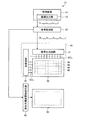•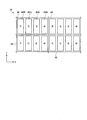•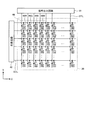•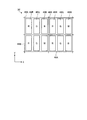•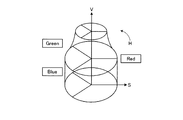•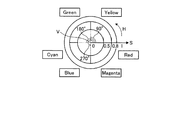•••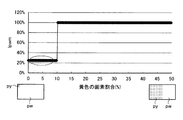••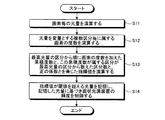•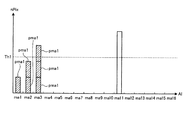•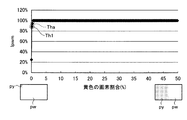•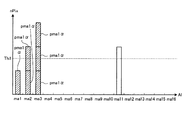•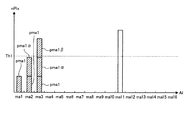••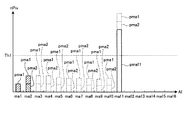•••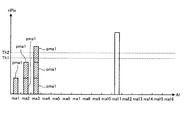•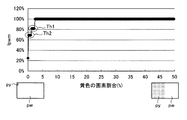•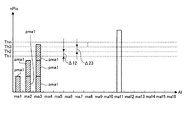•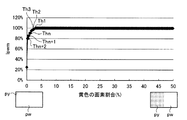••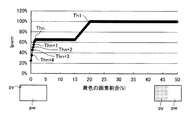•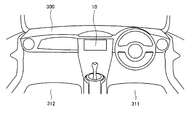•Classifications

• GPHYSICS
• G09EDUCATION; CRYPTOGRAPHY; DISPLAY; ADVERTISING; SEALS
• G09GARRANGEMENTS OR CIRCUITS FOR CONTROL OF INDICATING DEVICES USING STATIC MEANS TO PRESENT VARIABLE INFORMATION
• G09G5/00Control arrangements or circuits for visual indicators common to cathode-ray tube indicators and other visual indicators
• G09G5/10Intensity circuits
• GPHYSICS
• G09EDUCATION; CRYPTOGRAPHY; DISPLAY; ADVERTISING; SEALS
• G09GARRANGEMENTS OR CIRCUITS FOR CONTROL OF INDICATING DEVICES USING STATIC MEANS TO PRESENT VARIABLE INFORMATION
• G09G3/00Control arrangements or circuits, of interest only in connection with visual indicators other than cathode-ray tubes
• G09G3/20Control arrangements or circuits, of interest only in connection with visual indicators other than cathode-ray tubes for presentation of an assembly of a number of characters, e.g. a page, by composing the assembly by combination of individual elements arranged in a matrix no fixed position being assigned to or needed to be assigned to the individual characters or partial characters
• G09G3/34Control arrangements or circuits, of interest only in connection with visual indicators other than cathode-ray tubes for presentation of an assembly of a number of characters, e.g. a page, by composing the assembly by combination of individual elements arranged in a matrix no fixed position being assigned to or needed to be assigned to the individual characters or partial characters by control of light from an independent source
• G09G3/36Control arrangements or circuits, of interest only in connection with visual indicators other than cathode-ray tubes for presentation of an assembly of a number of characters, e.g. a page, by composing the assembly by combination of individual elements arranged in a matrix no fixed position being assigned to or needed to be assigned to the individual characters or partial characters by control of light from an independent source using liquid crystals
• G09G3/3607Control arrangements or circuits, of interest only in connection with visual indicators other than cathode-ray tubes for presentation of an assembly of a number of characters, e.g. a page, by composing the assembly by combination of individual elements arranged in a matrix no fixed position being assigned to or needed to be assigned to the individual characters or partial characters by control of light from an independent source using liquid crystals for displaying colours or for displaying grey scales with a specific pixel layout, e.g. using sub-pixels
• GPHYSICS
• G09EDUCATION; CRYPTOGRAPHY; DISPLAY; ADVERTISING; SEALS
• G09GARRANGEMENTS OR CIRCUITS FOR CONTROL OF INDICATING DEVICES USING STATIC MEANS TO PRESENT VARIABLE INFORMATION
• G09G3/00Control arrangements or circuits, of interest only in connection with visual indicators other than cathode-ray tubes
• G09G3/20Control arrangements or circuits, of interest only in connection with visual indicators other than cathode-ray tubes for presentation of an assembly of a number of characters, e.g. a page, by composing the assembly by combination of individual elements arranged in a matrix no fixed position being assigned to or needed to be assigned to the individual characters or partial characters
• G09G3/34Control arrangements or circuits, of interest only in connection with visual indicators other than cathode-ray tubes for presentation of an assembly of a number of characters, e.g. a page, by composing the assembly by combination of individual elements arranged in a matrix no fixed position being assigned to or needed to be assigned to the individual characters or partial characters by control of light from an independent source
• G09G3/36Control arrangements or circuits, of interest only in connection with visual indicators other than cathode-ray tubes for presentation of an assembly of a number of characters, e.g. a page, by composing the assembly by combination of individual elements arranged in a matrix no fixed position being assigned to or needed to be assigned to the individual characters or partial characters by control of light from an independent source using liquid crystals
• G09G3/3611Control of matrices with row and column drivers
• G09G3/3648Control of matrices with row and column drivers using an active matrix
• GPHYSICS
• G09EDUCATION; CRYPTOGRAPHY; DISPLAY; ADVERTISING; SEALS
• G09GARRANGEMENTS OR CIRCUITS FOR CONTROL OF INDICATING DEVICES USING STATIC MEANS TO PRESENT VARIABLE INFORMATION
• G09G5/00Control arrangements or circuits for visual indicators common to cathode-ray tube indicators and other visual indicators
• G09G5/02Control arrangements or circuits for visual indicators common to cathode-ray tube indicators and other visual indicators characterised by the way in which colour is displayed
• GPHYSICS
• G09EDUCATION; CRYPTOGRAPHY; DISPLAY; ADVERTISING; SEALS
• G09GARRANGEMENTS OR CIRCUITS FOR CONTROL OF INDICATING DEVICES USING STATIC MEANS TO PRESENT VARIABLE INFORMATION
• G09G2300/00Aspects of the constitution of display devices
• G09G2300/04Structural and physical details of display devices
• G09G2300/0439Pixel structures
• G09G2300/0452Details of colour pixel setup, e.g. pixel composed of a red, a blue and two green components
• GPHYSICS
• G09EDUCATION; CRYPTOGRAPHY; DISPLAY; ADVERTISING; SEALS
• G09GARRANGEMENTS OR CIRCUITS FOR CONTROL OF INDICATING DEVICES USING STATIC MEANS TO PRESENT VARIABLE INFORMATION
• G09G2340/00Aspects of display data processing
• G09G2340/04Changes in size, position or resolution of an image
• G09G2340/0457Improvement of perceived resolution by subpixel rendering
• GPHYSICS
• G09EDUCATION; CRYPTOGRAPHY; DISPLAY; ADVERTISING; SEALS
• G09GARRANGEMENTS OR CIRCUITS FOR CONTROL OF INDICATING DEVICES USING STATIC MEANS TO PRESENT VARIABLE INFORMATION
• G09G2340/00Aspects of display data processing
• G09G2340/06Colour space transformation
• GPHYSICS
• G09EDUCATION; CRYPTOGRAPHY; DISPLAY; ADVERTISING; SEALS
• G09GARRANGEMENTS OR CIRCUITS FOR CONTROL OF INDICATING DEVICES USING STATIC MEANS TO PRESENT VARIABLE INFORMATION
• G09G2360/00Aspects of the architecture of display systems
• G09G2360/16Calculation or use of calculated indices related to luminance levels in display data

Abstract

An appropriate output signal of a fourth sub-pixel that displays a fourth color component different from the first sub-pixel, the second sub-pixel, and the third sub-pixel is obtained and image quality deterioration of the display device is reduced. A display device receives input signals that can be displayed by a first subpixel, a second subpixel, and a third subpixel, and receives a first subpixel, a second subpixel, a third subpixel, and a fourth subpixel. And a signal processing unit that calculates each output signal to the sub-pixel. The signal processing unit calculates the amount of light of the planar light source necessary for each pixel, and calculates the frequency of the pixel to which it belongs for each of a plurality of categories with the amount of light of the obtained planar light source as a variable. And the signal processing unit counts the number of divisions counted from the highest light quantity category where the division to which the cumulative frequency is located, and the cumulative frequency obtained by adding the frequency of the pixel in order from the highest light quantity category required by the planar light source among at least the division And the brightness of the planar light source is controlled based on the amount of light of the planar light source that exceeds the threshold value. [Selection] Figure 1

Description

The present invention relates to a display device.

In recent years, the demand for display devices for mobile devices such as mobile phones and electronic paper has increased. In a display device, one pixel includes a plurality of sub-pixels, each of the plurality of sub-pixels outputs light of a different color, and the display of the sub-pixel is switched on and off, so that one pixel can perform various operations. The color is displayed. Such display devices have improved display characteristics such as resolution and luminance year by year. However, since the aperture ratio decreases as the resolution increases, there is a problem that, when trying to achieve high luminance, it is necessary to increase the luminance of the backlight, and the power consumption of the backlight increases. In order to improve this, there is a technique of adding a white pixel that is a fourth subpixel to the conventional red, green, and blue subpixels (for example, Patent Document 1 and Patent Document 2). This technology reduces the current value of the backlight and the power consumption by the amount that the white pixel improves the luminance.

JP 2012-108518 A JP 2011-1000014 A

Since the brightness of the backlight affects a plurality of pixels in the display unit, if the brightness of the backlight is reduced in accordance with the brightness of a specific pixel displayed by the input signal, other pixels should be displayed. There is a possibility that the luminance is insufficient and an appropriate color component cannot be displayed.

The present invention obtains an appropriate output signal of a fourth subpixel that displays a fourth color component different from the first subpixel, the second subpixel, and the third subpixel, and reduces image quality deterioration of the display device. Objective.

As one aspect of the present invention, a display device includes a first subpixel that displays a first color component, a second subpixel that displays a second color component, a third subpixel that displays a third color component, A display unit in which pixels including a first sub-pixel, a second sub-pixel, and a fourth sub-pixel that displays a fourth color component different from the third sub-pixel are arranged in a matrix; and the display unit And an input signal that can be displayed by the first subpixel, the second subpixel, and the third subpixel are input, and the first subpixel, the second subpixel, the first subpixel, A signal processing unit that calculates each output signal to three subpixels and the fourth subpixel, and the signal processing unit calculates a light amount of a planar light source required for each pixel, and the obtained Calculate the frequency of the pixel to which the light quantity of the surface light source is variable for each of the plurality of sections, and at least the surface light of the sections Calculating the index value obtained by multiplying the cumulative frequency obtained by adding the frequency of the pixels in order from the maximum light amount required by the number of segments counted from the maximum light amount category where the category to which the cumulative frequency belongs is located, The brightness of the planar light source is controlled based on the light amount of the planar light source that exceeds the threshold value.

As another aspect of the present invention, a display device includes a first subpixel that displays a first color component, a second subpixel that displays a second color component, and a third subpixel that displays a third color component. A display unit in which pixels including a first sub-pixel, a second sub-pixel that displays a fourth color component different from the third sub-pixel, and a fourth sub-pixel that displays a fourth color component are arranged in a matrix, and the display A planar light source that irradiates a portion, and an input signal that can be displayed by the first subpixel, the second subpixel, and the third subpixel, and the first subpixel, the second subpixel, A signal processing unit that calculates output signals to the third sub-pixel and the fourth sub-pixel, and the signal processing unit is obtained by calculating a light amount of the planar light source necessary for each pixel. In addition, the frequency of the pixels belonging to each of the plurality of categories with the light quantity of the surface light source as a variable is calculated, and the highest light quantity of the sections is calculated. A cumulative frequency obtained by adding the frequency of the pixels in order, and an index value for each category, the cumulative frequency of the category to be determined, and a category for determining the index value of the category on the side of the maximum light quantity from the required category The index value obtained by adding the value multiplied by the positive coefficient determined in (5) is calculated for each of the sections, and the luminance of the planar light source is controlled based on the section where the index value exceeds the threshold.

FIG. 1 is a block diagram illustrating an example of a configuration of a display device according to the present embodiment. FIG. 2 is a diagram showing a pixel array of the image display panel according to the present embodiment. FIG. 3 is a conceptual diagram of an image display panel and an image display panel driving circuit of the display device according to the present embodiment. FIG. 4 is a diagram illustrating another example of the pixel array of the image display panel according to the present embodiment. FIG. 5 is a conceptual diagram of a reproduction HSV color space that can be reproduced by the display device of this embodiment. FIG. 6 is a conceptual diagram showing the relationship between the hue and saturation of the reproduction HSV color space. FIG. 7 is an example of the frequency distribution of the input signal. FIG. 8 is an explanatory diagram for explaining the cumulative frequency distribution of FIG. FIG. 9 is an explanatory diagram for explaining an example in which the replacement ratio of the fourth sub-pixel is greatly changed at a specific pixel ratio depending on a predetermined threshold. FIG. 10 is an explanatory diagram for explaining an example in which the replacement ratio of the fourth sub-pixel changes greatly at a specific pixel ratio depending on a predetermined threshold. FIG. 11 is a flowchart for explaining the processing procedure of the color conversion processing according to the present embodiment. FIG. 12 is an explanatory diagram for explaining the relationship between the index value and the threshold according to the present embodiment. FIG. 13 is an explanatory diagram for explaining the replacement ratio of the fourth sub-pixel in the present embodiment. FIG. 14 is an explanatory diagram for explaining another example of the relationship between the index value and the threshold according to the present embodiment. FIG. 15 is an explanatory diagram for explaining another example of the relationship between the index value and the threshold according to the present embodiment. FIG. 16 is an example of the frequency distribution of the input signal. FIG. 17 is an explanatory diagram for explaining the cumulative frequency distribution of FIG. FIG. 18 is an explanatory diagram for explaining the relationship between the index value and the threshold according to the present embodiment. FIG. 19 is an example of the frequency distribution of the input signal. FIG. 20 is an example of the frequency distribution of the input signal. FIG. 21 is an explanatory diagram for explaining the replacement ratio of the fourth sub-pixel with the two-stage threshold according to the present embodiment. FIG. 22 is an example of the frequency distribution of the input signal. FIG. 23 is an explanatory diagram for explaining the replacement ratio of the fourth sub-pixel with the multi-stage threshold according to the present embodiment. FIG. 24 is an example of the frequency distribution of the input signal. FIG. 25 is an explanatory diagram for describing the replacement ratio of the fourth sub-pixel with the multi-stage threshold according to the present embodiment. FIG. 26 is a diagram illustrating an example of an electronic apparatus including the display device according to this embodiment. FIG. 27 is a diagram illustrating an example of an electronic apparatus including the display device according to this embodiment.

EMBODIMENT OF THE INVENTION About the form (embodiment) for inventing, it demonstrates in detail, referring drawings. The present invention is not limited by the contents described in the following embodiments. The constituent elements described below include those that can be easily assumed by those skilled in the art and those that are substantially the same. Furthermore, the constituent elements described below can be appropriately combined. It should be noted that the disclosure is merely an example, and those skilled in the art can easily conceive of appropriate modifications while maintaining the gist of the invention are naturally included in the scope of the present invention. In addition, the drawings may be schematically represented with respect to the width, thickness, shape, and the like of each part in comparison with actual aspects for the sake of clarity of explanation, but are merely examples, and the interpretation of the present invention is not limited. It is not limited. In addition, in the present specification and each drawing, elements similar to those described above with reference to the previous drawings are denoted by the same reference numerals, and detailed description may be omitted as appropriate.

FIG. 1 is a block diagram illustrating an example of a configuration of a display device according to the present embodiment. FIG. 2 is a diagram showing a pixel array of the image display panel according to the present embodiment. FIG. 3 is a conceptual diagram of an image display panel and an image display panel driving circuit of the display device according to the present embodiment. FIG. 4 is a diagram illustrating another example of the pixel array of the image display panel according to the present embodiment.

As shown in FIG. 1, the display device 10 includes a signal processing unit 20 that receives an input signal (RGB data) from the image output unit 12 of the control device 11, performs predetermined data conversion processing, and outputs the signal processing unit 20. An image display panel (display unit) 30 that displays an image based on an output signal output from the unit 20, an image display panel drive circuit 40 that controls driving of the image display panel 30, and illumination of the image display panel 30 from the back side And a planar light source device control circuit 60 for controlling the driving of the planar light source device 50. The display device 10 has the same configuration as the image display device assembly described in JP 2011-154323 A, and various modifications described in JP 2011-154323 A can be applied. is there.

The signal processing unit 20 is an arithmetic processing unit that controls operations of the image display panel 30 and the planar light source device 50. The signal processing unit 20 is connected to an image display panel drive circuit 40 for driving the image display panel 30 and a planar light source device control circuit 60 for driving the planar light source device 50. The signal processing unit 20 processes an input signal input from the outside to generate an output signal and a planar light source device control signal. That is, the signal processing unit 20 reproduces the input HSV color space input value (input signal) of the input signal with the first color, the second color, the third color, and the fourth color component. A color space reproduction value (output signal) is generated by conversion, and the generated output signal is output to the image display panel 30. The signal processing unit 20 outputs the generated output signal to the image display panel drive circuit 40, and outputs the generated planar light source device control signal to the planar light source device control circuit 60.

As shown in FIGS. 2 and 3, the image display panel 30 has pixels 48 arranged in a two-dimensional matrix with P 0 × Q 0 (P 0 in the row direction and Q 0 in the column direction). ing. 2 and 3 show an example in which a plurality of pixels 48 are arranged in a matrix in an XY two-dimensional coordinate system. In this example, the row direction is the X direction and the column direction is the Y direction.

The pixel 48 includes a first sub pixel 49R, a second sub pixel 49G, a third sub pixel 49B, and a fourth sub pixel 49W. The first sub-pixel 49R displays a first color component (for example, red as the first primary color). The second subpixel 49G displays a second color component (for example, green as the second primary color). The third sub-pixel 49B displays a third color component (for example, blue as the third primary color). The fourth subpixel 49W displays a fourth color component (for example, white). Hereinafter, the first sub-pixel 49R, the second sub-pixel 49G, the third sub-pixel 49B, and the fourth sub-pixel 49W are referred to as sub-pixels 49 when it is not necessary to distinguish them from each other. The image output unit 12 described above outputs RGB data that can be displayed by the first color component, the second color component, and the third color component in the pixel 48 as an input signal of the signal processing unit 20.

More specifically, the display device 10 is a transmissive color liquid crystal display device. The image display panel 30 is a color liquid crystal display panel, and a first color filter that passes the first primary color is disposed between the first sub-pixel 49R and the image observer, and the second sub-pixel 49G, the image observer, A second color filter that allows the second primary color to pass therethrough is disposed, and a third color filter that allows the third primary color to pass is disposed between the third sub-pixel 49B and the image observer. In the image display panel 30, no color filter is disposed between the fourth sub-pixel 49W and the image observer. The fourth subpixel 49W may be provided with a transparent resin layer instead of the color filter. As described above, by providing the transparent resin layer, the image display panel 30 can suppress the occurrence of a large step in the fourth subpixel 49W by not providing the color filter in the fourth subpixel 49W.

In the example shown in FIG. 2, the image display panel 30 includes the first sub-pixel 49R, the second sub-pixel 49G, the third sub-pixel 49B, and the fourth sub-pixel 49W arranged in an arrangement similar to the stripe arrangement. . Note that the structure and arrangement of the sub-pixels 49R, 49G, 49B, and 49W included in one pixel 48 are not particularly limited. For example, in the image display panel 30, the first sub-pixel 49R, the second sub-pixel 49G, the third sub-pixel 49B, and the fourth sub-pixel 49W may be arranged in an arrangement similar to a diagonal arrangement (mosaic arrangement). Further, for example, an array similar to a delta array (triangle array), an array similar to a rectangle array, or the like may be used. Further, as in the image display panel 30 ′ shown in FIG. 4, a pixel 48A having a first subpixel 49R, a second subpixel 49G, and a third subpixel 49B, a first subpixel 49R, a second subpixel 49G, and The pixels 48B having the fourth sub-pixels 49W may be alternately arranged in the row direction and the column direction.

In general, an array similar to the stripe array is suitable for displaying data and character strings on a personal computer or the like. On the other hand, an arrangement similar to a mosaic arrangement is suitable for displaying a natural image on a video camera recorder or a digital still camera.

The image display panel drive circuit 40 includes a signal output circuit 41 and a scanning circuit 42. The image display panel drive circuit 40 holds the video signal by the signal output circuit 41 and sequentially outputs it to the image display panel 30. The signal output circuit 41 is electrically connected to the image display panel 30 through a wiring DTL. In the image display panel drive circuit 40, the scanning circuit 42 controls on / off of a switching element (for example, TFT) for controlling the operation (light transmittance) of the sub-pixel in the image display panel 30. The scanning circuit 42 is electrically connected to the image display panel 30 through a wiring SCL.

The planar light source device 50 is disposed on the back surface of the image display panel 30 and illuminates the image display panel 30 by irradiating light toward the image display panel 30. The planar light source device 50 irradiates light on the entire surface of the image display panel 30 to brighten the image display panel 30. The planar light source device control circuit 60 controls the amount of light output from the planar light source device 50. Specifically, the planar light source device control circuit 60 generates a current supplied to the planar light source device 50 based on the planar light source device control signal output from the signal processing unit 20 by PWM (Pulse Width Modulation) or the like. Is adjusted to adjust the output power amount of the planar light source device 50 (corresponding to the light source power amount below). Thereby, the light quantity (light intensity) of the light which irradiates the image display panel 30 is controlled.

FIG. 5 is a conceptual diagram of a reproduction HSV color space that can be reproduced by the display device of this embodiment. FIG. 6 is a conceptual diagram showing the relationship between the hue and saturation of the reproduction HSV color space. The signal processing unit 20 receives an input signal that is information on an image to be displayed from the outside. The input signal includes information on an image (color) to be displayed at that position for each pixel 48 as an input signal. Specifically, in the image display panel 30 in which P 0 × Q 0 pixels 48 are arranged in a matrix, the (p, q) -th pixel 48 (where 1 ≦ p ≦ P 0 , 1 ≦ q ≦ Q 0 ), the input signal of the first subpixel 49R whose signal value is x 1− (p, q) , the input signal of the second subpixel 49G whose signal value is x 2− (p, q) , and A signal including an input signal (see FIG. 1 ) of the third subpixel 49B having a signal value of x 3- (p, q) is input to the signal processing unit 20.

The signal processing unit 20 illustrated in FIG. 1 processes the input signal, thereby outputting an output signal (signal value X 1- (p, q) of the first sub-pixel for determining the display gradation of the first sub-pixel 49R. ), An output signal of the second subpixel (signal value X 2-(p, q) ) for determining the display gradation of the second subpixel 49G, and a display gradation of the third subpixel 49B. The output signal of the third subpixel (signal value X 3- (p, q) ) and the output signal of the fourth subpixel for determining the display gradation of the fourth subpixel 49W (signal value X 4- (p, q) ) is generated and output to the image display panel drive circuit 40.

The display device 10 includes the fourth sub-pixel 49W that outputs a fourth color component (for example, white) to the pixel 48, so that the dynamic range of lightness in the HSV color space (reproduction HSV color space) as illustrated in FIG. Can be spread. That is, as shown in FIG. 5, in the columnar HSV color space that can be displayed by the first sub-pixel 49R, the second sub-pixel 49G, and the third sub-pixel 49B, the maximum brightness V increases as the saturation S increases. It becomes a shape on which a solid body having a substantially trapezoidal shape with a low value is placed.

By adding the fourth color component (white), the signal processing unit 20 stores the maximum value Vmax (S) of brightness with the saturation S in the enlarged HSV color space as a variable stored in the signal processing unit 20. Yes. That is, the signal processing unit 20 stores the value of the maximum brightness value Vmax (S) for each coordinate (value) of saturation and hue with respect to the three-dimensional shape of the HSV color space shown in FIG. Since the input signal includes the input signals of the first sub-pixel 49R, the second sub-pixel 49G, and the third sub-pixel 49B, the HSV color space of the input signal has a cylindrical shape, that is, a cylindrical portion of the reproduction HSV color space. It becomes the same shape.

Next, the signal processor 20 outputs an output signal (signal value X) of the first subpixel 49R based on at least the input signal (signal value x1- (p, q) ) of the first subpixel 49R and the expansion coefficient α. 1- (p, q) ) is calculated and output to the first sub-pixel 49R. Further, the signal processing unit 20 outputs at least the output signal (signal value X 2− ) of the second sub-pixel 49G based on the input signal (signal value x 2− (p, q) ) and the expansion coefficient α of the second sub-pixel 49G. (P, q) ) is calculated and output to the second sub-pixel 49G. The signal processing unit 20 has at least an input signal (signal value x 3- (p, q)) of the third sub-pixel 49B and the output signal of the third sub-pixel 49B based on the expansion coefficient alpha (signal value X 3- (P, q) ) is calculated and output to the third sub-pixel 49B. Further, the signal processing unit 20, an input signal of the first sub-pixel 49R (signal value x 1- (p, q)) , the input signal of the second sub-pixel 49G (signal value x 2- (p, q)) and Based on the input signal (signal value x 3-(p, q) ) of the third sub-pixel 49B, the output signal (signal value X 4- (p, q) ) of the fourth sub-pixel 49W is calculated , and the fourth sub-pixel is calculated. Output to the pixel 49W.

Specifically, the signal processing unit 20 calculates the output signal of the first subpixel 49R based on the expansion coefficient α of the first subpixel 49R and the output signal of the fourth subpixel 49W, and outputs the second subpixel 49G. An output signal of the second subpixel 49G is calculated based on the expansion coefficient α and the output signal of the fourth subpixel 49W, and a third signal is calculated based on the expansion coefficient α of the third subpixel 49B and the output signal of the fourth subpixel 49W. An output signal of the sub-pixel 49B is calculated.

That is, the signal processing unit 20 sets (p, q) -th pixel (or first sub-pixel 49R, second sub-pixel 49G, and third sub-pixel 49B as a set when χ is a constant depending on the display device. ), The signal value X 1- (p, q) that is the output signal of the first sub-pixel 49R, the signal value X 2- (p, q) that is the output signal of the second sub-pixel 49G, and the third sub-pixel 49B. The signal value X 3- (p, q) that is the output signal of is obtained from the following equations (1) to (3).
X1- (p, q) = [alpha] .x1- (p, q)-[ chi] .X4- (p, q) (1)
X2- (p, q) = [alpha] .x2- (p, q)-[ chi] .X4- (p, q) (2)
X 3-(p, q) = α · x 3-(p, q) -χ · X 4-(p, q) (3)

The signal processing unit 20 obtains the maximum value Vmax (S) of the brightness with the saturation S in the HSV color space expanded by adding the fourth color component as a variable, and the input signal of the sub-pixel 49 in the plurality of pixels 48. Based on the values, the saturation S and the lightness V (S) in the plurality of pixels 48 are obtained.

Saturation S and lightness V (S) are represented by S = (Max−Min) / Max and V (S) = Max. The saturation S can take a value from 0 to 1, and the lightness V (S) can take a value from 0 to (2 n −1). n is the number of display gradation bits. Further, Max is the maximum value among the input signal value of the first sub-pixel 49R, the input signal value of the second sub-pixel 49G, and the input signal value of the third sub-pixel 49B to the pixel 48. Min is the minimum value among the input signal value of the first sub-pixel 49R, the input signal value of the second sub-pixel 49G, and the input signal value of the third sub-pixel 49B to the pixel 48. Further, the hue H is represented by 0 ° to 360 ° as shown in FIG. From 0 ° to 360 °, the colors are red (Red), yellow (Yellow), green (Green), cyan (Cyan), blue (Blue), magenta (Magenta), and red.

In the present embodiment, the signal value X 4- (p, q) can be obtained based on the product of Min (p, q) and the expansion coefficient α. Specifically, the signal value X 4- (p, q) can be obtained based on the following equation (4). In Expression (4), the product of Min (p, q) and the expansion coefficient α is divided by χ, but the present invention is not limited to this. χ will be described later. Further, the expansion coefficient α is determined for each image display frame.
X 4− (p, q) = Min (p, q) · α / χ (4)

Generally, in the (p, q) th pixel, the input signal of the first sub-pixel 49R (signal value x 1- (p, q)) , the input signal of the second sub-pixel 49G (signal value x 2-(p , Q) ) and the input signal (signal value x3- (p, q) ) of the third sub-pixel 49B, saturation S (p, q) and brightness in the HSV color space of the cylinder V (S) (p, q) can be obtained from the following equations (5) and (6).
S (p, q) = (Max (p, q) −Min (p, q) ) / Max (p, q) (5)
V (S) (p, q) = Max (p, q) (6)

Here, Max (p, q) is an input signal value of three sub-pixels 49 of (x 1- (p, q) , x 2- (p, q) , x 3- (p, q) ). Min (p, q) is the value of three subpixels 49 of (x 1-(p, q) , x 2-(p, q) , x 3-(p, q) ) This is the minimum value of the input signal value. In this embodiment, n = 8. That is, the number of display gradation bits is 8 bits (the display gradation value is 256 gradations from 0 to 255).

No color filter is arranged in the fourth sub-pixel 49W that displays white. A signal having a value corresponding to the maximum signal value of the output signal of the first subpixel is input to the first subpixel 49R, and a value corresponding to the maximum signal value of the output signal of the second subpixel is input to the second subpixel 49G. The first subpixel included in the pixel 48 or the group of pixels 48 when a signal having a value corresponding to the maximum signal value of the output signal of the third subpixel is input to the third subpixel 49B. The luminance of the assembly of 49R, the second subpixel 49G, and the third subpixel 49B is BN 1-3 . The luminance of the fourth subpixel 49W when a signal having a value corresponding to the maximum signal value of the output signal of the fourth subpixel 49W is input to the fourth subpixel 49W included in the pixel 48 or the group of pixels 48. Assume that BN 4 is set. That is, the maximum luminance white is displayed by the aggregate of the first sub-pixel 49R, the second sub-pixel 49G, and the third sub-pixel 49B, and this white luminance is represented by BN 1-3 . Then, when χ is a constant depending on the display device, the constant χ is represented by χ = BN 4 / BN 1-3 .

Specifically, a signal value x 1− (p, q) is input to the aggregate of the first subpixel 49R, the second subpixel 49G, and the third subpixel 49B as an input signal having the next display gradation value. = 255, relative to the signal value x 2- (p, q) = 255, the white luminance BN 1-3 at the time when the signal value x 3- (p, q) = 255 is input, the fourth subpixel 49W For example, the luminance BN 4 when the input signal having the display gradation value 255 is input is 1.5 times. That is, in this embodiment, χ = 1.5.

By the way, when the signal value X 4- (p, q) is given by the above-described equation (4), Vmax (S) can be expressed by the following equations (7) and (8).
If S ≦ S 0 ,
Vmax (S) = (χ + 1) · (2 n −1) (7)
If S 0 <S ≦ 1,
Vmax (S) = (2 n −1) · (1 / S) (8)
Here, S 0 = 1 / (χ + 1).

The maximum brightness value Vmax (S) obtained by adding the fourth color component in the HSV color space and using the saturation S as a variable is, for example, a kind of look for the signal processing unit 20. • Stored as an up table. Alternatively, the maximum value Vmax (S) of lightness with the saturation S in the enlarged HSV color space as a variable is obtained in the signal processing unit 20 each time.

Next, signal values X 1- (p, q) , X 2- (p, q) , X 3- (p, q) , X 4− which are output signals at the (p, q) -th pixel 48. A method for obtaining (p, q) (extension processing) will be described. The next processing is the luminance of the first primary color displayed by (first subpixel 49R + fourth subpixel 49W), the luminance of the second primary color displayed by (second subpixel 49G + fourth subpixel 49W), ( This is performed so as to maintain the luminance ratio of the third primary color displayed by the third subpixel 49B + the fourth subpixel 49W). In addition, the color tone is maintained (maintained). Furthermore, the gradation-luminance characteristics (gamma characteristics, γ characteristics) are maintained (maintained). Further, when any of the input signal values is zero or small in any one of the pixels 48 or the group of pixels 48, the expansion coefficient α may be obtained without including such a pixel 48 or the group of pixels 48. .

(First step)
First, the signal processing unit 20 obtains the saturation S and the lightness V (S) in the plurality of pixels 48 based on the input signal values of the sub-pixels 49 in the plurality of pixels 48. Specifically, a signal value x 1− (p, q) that is an input signal of the first subpixel 49R to the (p, q) th pixel 48, and a signal value that is an input signal of the second subpixel 49G. Based on x 2− (p, q) , signal value x 3− (p, q) that is an input signal of the third sub-pixel 49B, S (p, q) , V from Formula (5) and Formula (6) (S) Find (p, q) . The signal processing unit 20 performs this process for all the pixels 48.

(Second step)
Next, the signal processing unit 20 obtains the expansion coefficient α (S) based on Vmax (S) / V (S) obtained in the plurality of pixels 48.

α (S) = Vmax (S) / V (S) (9)

(Third step)
Next, the signal processing unit 20 uses the signal value X 4− (p, q) in the (p, q) -th pixel 48 as at least the signal value x 1− (p, q) of the input signal and the signal value. It calculates | requires based on x2- (p, q) and signal value x3- (p, q) . In the present embodiment, the signal processing unit 20 determines the signal value X 4- (p, q) based on Min (p, q) , the expansion coefficient α, and the constant χ. More specifically, as described above, the signal processing unit 20 obtains the signal value X 4- (p, q) based on the above equation (4). The signal processing unit 20 obtains a signal value X 4− (p, q) in all of the P 0 × Q 0 pixels 48.

(4th process)
Thereafter, the signal processing unit 20 converts the signal value X 1- (p, q) in the (p, q) -th pixel 48 into the signal value x 1- (p, q) , the expansion coefficient α, and the signal value X 4. - (p, q) obtained based on, the (p, q) th pixel 48 the signal value X 2 in - (p, q) and the signal value x 2 - (p, q) , expansion coefficient α and the signal value X 4- (p, q) , the signal value X 3- (p, q) at the (p, q) -th pixel 48 is converted into the signal value x 3- (p, q) , the expansion coefficient α, and Obtained based on the signal value X 4− (p, q) . Specifically, the signal processing unit 20 outputs the signal value X 1- (p, q) , the signal value X 2- (p, q), and the signal value X 3- ( p, q) is obtained based on the above formulas (1) to (3).

The signal processing unit 20 extends the value of Min (p, q) by α as shown in the equation (4). As described above, the value of Min (p, q) is expanded by α, so that not only the luminance of the white display subpixel (the fourth subpixel 49W) increases but also the red display subpixel as shown in the above formula. The luminance of the pixel, the green display subpixel, and the blue display subpixel (corresponding to the first subpixel 49R, the second subpixel 49G, and the third subpixel 49B, respectively) also increases. For this reason, the problem that the dullness of color generate | occur | produces can be avoided. That is, as compared with the case where the value of Min (p, q) is not extended, that the value of Min (p, q) is extended by alpha, luminance image as a whole becomes alpha times. Therefore, for example, an image display such as a still image can be performed with high luminance, which is preferable.

Displayed by output signals X 1- (p, q) , X 2- (p, q) , X 3- (p, q) , X 4- (p, q) at the (p, q) -th pixel 48 The luminance is expanded to α times the luminance formed from the input signals x 1- (p, q) , x 2− (p, q) , x 3− (p, q) . For this reason, the display device 10 may reduce the luminance of the planar light source device 50 based on the expansion coefficient α in order to obtain the same pixel luminance as that of the pixel 48 that has not been expanded. Specifically, the brightness of the planar light source device 50 may be (1 / α) times.

(Determination of light intensity of the planar light source device)
As described above, the planar light source device control circuit 60 generates a current supplied to the planar light source device 50 based on the planar light source device control signal output from the signal processing unit 20 by PWM (Pulse Width Modulation) or the like. Is adjusted to adjust the output power amount of the planar light source device 50 (corresponding to the light source power amount below). Thereby, the light quantity (light intensity) of the light which irradiates the image display panel (display unit) 30 is controlled. For this reason, the control amount adjusted by PWM is proportional to (1 / α) described above. FIG. 7 is an example of the frequency distribution of the input signal. FIG. 8 is an explanatory diagram for explaining the cumulative frequency distribution of FIG. 9 and 10 are explanatory diagrams for explaining an example in which the replacement ratio of the fourth sub-pixel is greatly changed at a specific pixel ratio depending on a predetermined threshold value. With reference to FIGS. 7 to 10, a case where the input signal displays yellow in some of all the pixels of the image display panel (display unit) 30 and white in the remaining pixels will be described.

As shown in FIG. 7, the signal processing unit 20 uses a light quantity Al (light intensity) of light that irradiates the image display panel (display unit) 30 as a variable, and a plurality of sections (for example, sections equally divided into 16 sections) ma1. The frequency nPix of the pixel to which it belongs is calculated for each section of ~ ma16. By this calculation, the pixel py (see FIG. 9) that displays yellow is counted in the section ma1, and the pixel pw (see FIG. 9) that displays white is counted in the section ma11. The section ma1 is a section with the largest light amount with which the planar light source device 50 emits the largest amount of light. The light quantity Al can be suppressed in order of the section ma2, the section ma3,. The signal processing unit 20 stores in advance a threshold value Th1 that determines the light amount Al of light that irradiates the image display panel (display unit) 30. In the cumulative frequency distribution shown in FIG. 8, the light amount of the section that exceeds the threshold value Th1. The planar light source device 50 is controlled by PWM so as to be Al.

In the cumulative frequency distribution shown in FIG. 8, the pixel number pma1 in which the pixel py (see FIG. 9) displaying yellow is counted in the section ma1, and the pixel pw (see FIG. 9) displaying white is counted in the section ma11. It is calculated only with the pixel number pma11. When the pixel number pma1 does not exceed the threshold Th1, the cumulative frequency is the pixel number pma1 from the division ma2 to the division ma10. For this reason, since the cumulative frequency exceeds the threshold Th1 for the first time in the section pma11, the signal processing unit 20 performs PWM control on the planar light source device 50 so that the amount of light Al corresponds to the section ma11.

As shown in FIG. 9, the display device 10 displays the brightness of the pixel 48 that is displaying white and displaying only the first subpixel, the second subpixel, and the third subpixel (in an unexpanded state). In order to obtain the luminance of the same pixel, the replacement ratio of the fourth subpixel can be increased. As a result, the planar light source device 50 can reduce the light source power amount lpwm (for example, about 20% in FIG. 9) based on the expansion coefficient α that becomes the light amount Al. When the luminance of the backlight is reduced in accordance with a specific pixel displayed by the input signal, that is, a pixel pw in which white is displayed, a pixel py that displays yellow that other pixels should have displayed (see FIG. 9). ) May be insufficient, and an appropriate color component may not be displayed.

When the ratio of the pixel py that displays yellow to the pixel that displays the pixel pw that displays white (hereinafter referred to as the yellow pixel ratio) is increased, the signal processing is performed when the number of pixels pma1 in the section ma1 exceeds the threshold in FIG. The unit 20 performs PWM control on the planar light source device 50 so that the amount of light Al corresponds to the section ma1. Yellow has high human visibility and it is difficult to increase the replacement ratio of the fourth sub-pixel, so the light source power lpwm must be increased. For this reason, as shown in FIG. 9, the light source power amount lpwm largely fluctuates (for example, changes from 20% to 100%) in a specific yellow pixel ratio. For example, when the ratio of the pixel py that displays yellow with respect to the pixel pw that displays white changes significantly in the input signal image, the light source power amount lpwm, that is, the replacement ratio of the fourth sub-pixel before and after the change. Since it changes suddenly, there is a possibility that the yellow color with high visibility changes. In the present embodiment, yellow is described as an example, but it is necessary to suppress a change in color also in the region from yellow (Yellow) to red (Red) shown in FIG. Regardless of the hue, the color space in the high-saturation region (for example, the saturation S shown in FIG. 6 is 0.8 or more) is also easily affected by the change in the replacement ratio of the fourth subpixel. As shown in FIG. 8, by lowering the threshold value Th1 so as to be the threshold value Th2, the threshold value Th2 is exceeded even in the section ma1, and the change in the replacement ratio of the fourth subpixel can be suppressed. However, as shown in FIG. 10, when the threshold value Th1 is lowered and the threshold value is Th2, the light source power amount lpwm stays high and power consumption cannot be suppressed.

FIG. 11 is a flowchart for explaining the processing procedure of the color conversion processing according to the present embodiment. FIG. 12 is an explanatory diagram for explaining the relationship between the index value and the threshold according to the present embodiment. FIG. 13 is an explanatory diagram for explaining the replacement ratio of the fourth sub-pixel in the present embodiment. With reference to FIGS. 7, 8, 9, 10, 11, 12, and 13, a color conversion method that can suppress image quality degradation while suppressing power consumption will be described.

As shown in FIG. 11, the signal processing unit 20 performs the operations of the first process to the second process described above, obtains the expansion coefficient α for each pixel 48, and obtains the optimum light amount for each pixel 48 (step S11). ).

Next, the signal processing unit 20 uses a light quantity Al (light intensity) of light that irradiates the image display panel (display unit) 30 as a variable, and each of a plurality of sections (for example, sections equally divided into 16 sections) ma1 to ma16. For each division, the frequency nPix of the pixel to which it belongs is calculated (step S12). The signal processing unit 20 stores a frequency distribution as shown in FIG.

Next, the signal processing unit 20 calculates the cumulative power obtained by adding the pixel power nPix in order from the highest light quantity section ma1. For example, the cumulative frequency distribution is as shown in FIG. Then, the signal processing unit 20 calculates an index value by multiplying the number of sections counted by the section to which the cumulative frequency belongs from the section with the highest light amount by a coefficient k (k is a positive arbitrary value) and further multiplying the cumulative frequency. (Step S13). For example, the coefficient k is any value of k = 0.5, 1, 1.5, 2, 2.5, and 3. However, the value of the coefficient k is an example, and a different value may be used depending on the category. First, the case where the coefficient k is k = 1 will be described below.

The signal processing unit 20 calculates the index value in order from the highest light quantity section ma1. For example, as shown in FIG. 12, in the section ma1, the number of pixels pma1 in which the pixel py (see FIG. 13) displaying yellow is counted in the section ma1, the number of sections 1 counted from the section ma1 with the highest light quantity, and the coefficient k And 1 × pma1 × k = pma1. Next, in the section ma2, the pixel py (see FIG. 13) that displays yellow is multiplied by the number of pixels pma1 counted in the section ma1, the number of sections 2 counted from the section ma1 with the highest light quantity, and the coefficient k to be 2 Xpma1 * k = 2 * pma1. In the section ma3, 3 × pma1 is obtained by multiplying the number of pixels pma1 in which the pixel py (see FIG. 13) displaying yellow is counted in the section ma1, the number of sections 3 counted from the section ma1 with the highest light quantity, and the coefficient k. Xk = 3 * pma1. The signal processing unit 20 stores the light amount Al corresponding to the section ma3 in which the obtained index value exceeds the threshold Th1, and the signal processing unit 20 outputs the light amount so that the light amount Al corresponding to the stored section ma3 is obtained. Are adjusted, and the planar light source device 50 is PWM-controlled (step S14). Thereby, the signal processing unit 20 can control the luminance of the planar light source device 50.

In step S13, the signal processing unit 20 may omit the multiplication of the coefficient k when k = 1. For example, as shown in FIG. 12, in the section ma1, the pixel py (see FIG. 13) displaying yellow is multiplied by the number of pixels pma1 counted in the section ma1 and the number of sections 1 counted from the section ma1 with the highest light amount, pma1. Next, in the section ma2, the pixel py (see FIG. 13) that displays yellow is multiplied by the number of pixels pma1 counted in the section ma1 and the number of sections 2 counted from the section ma1 with the highest light amount to be 2 × pma1. In the section ma3, the pixel py (see FIG. 13) displaying yellow is multiplied by the number of pixels pma1 counted in the section ma1 and the number of sections 3 counted from the section ma1 with the highest light amount to be 3 × pma1. The signal processing unit 20 stores the light amount Al corresponding to the section ma3 in which the obtained index value exceeds the threshold Th1, and the signal processing unit 20 outputs the light amount so that the light amount Al corresponding to the stored section ma3 is obtained. Are adjusted, and the planar light source device 50 is PWM-controlled (step S14).

As illustrated in FIG. 13, the signal processing unit 20 can reduce power with the light amount Al corresponding to the section ma3. Therefore, image quality degradation can be suppressed while suppressing power even in a region where the yellow pixel ratio is low.

In step S13, the signal processing unit 20 has obtained an example in which the number of divisions n × the number of pixels pma1 × the coefficient k is shown. However, the present invention is not limited to this. For example, the signal processing unit 20 calculates the index value in order from the highest light quantity section ma1. For example, as shown in FIG. 12, in the section ma1, the pixel py (see FIG. 13) displaying yellow is counted by the number of pixels pma1 counted in the section ma1, and the accumulation on the side of the section ma1 with the highest light quantity is higher than the section ma1 with the highest light quantity. Since the number of pixels as the frequency is 0, the index value becomes pma1 + 0 × k = pma1 when the value obtained by multiplying 0 by the coefficient k is added. Next, in the division ma2, the number of pixels pma1 that is the cumulative frequency, the index value pma1 of the division ma1 that is on the side of the division with the highest light quantity than the desired division, and the positive coefficient k (= 1) determined in the division ma2 are multiplied. An index value obtained by adding the calculated value pma1 × k is pma1 + pma1 × 1 = 2 × pma1. In the division ma3, the pixel number pma1 which is the cumulative frequency, the index value 2 × pma1 of the division ma2 on the side of the division with the highest light quantity than the division ma3 to be obtained, and the positive coefficient k (= 1) determined in the division ma2. An index value obtained by adding the multiplied value pma1 × k is pma1 + 2 × pma1 × 1 = 3 × pma1. The pixel py (see FIG. 13) displaying yellow is multiplied by the number of pixels pma1 counted in the section ma3, the number of sections 3 counted from the section ma1 with the highest light quantity, and the coefficient k, and 3 × pma1 × k = 3 Xpma1. The signal processing unit 20 stores the light amount Al corresponding to the section ma3 in which the obtained index value exceeds the threshold Th1, and the signal processing unit 20 outputs the light amount so that the light amount Al corresponding to the stored section ma3 is obtained. Are adjusted, and the planar light source device 50 is PWM-controlled (step S14). Thereby, the signal processing unit 20 can control the luminance of the planar light source device 50.

In the above, assuming that the coefficient k = 1, the signal processing unit 20 calculates the index value by multiplying the number of sections that the section to which the cumulative frequency belongs from the section with the highest light quantity by the coefficient k and further multiplying by the cumulative power. However, the present embodiment is not limited to this case. FIG. 14 is an explanatory diagram for explaining another example of the relationship between the index value and the threshold according to the present embodiment.

First, as shown in FIG. 11, the signal processing unit 20 performs the operations of the first process to the second process described above, obtains the expansion coefficient α for each pixel 48, and obtains the optimum light amount for each pixel 48 ( Step S11). Next, the signal processing unit 20 uses a light quantity Al (light intensity) of light that irradiates the image display panel (display unit) 30 as a variable, and each of a plurality of sections (for example, sections equally divided into 16 sections) ma1 to ma16. For each division, the frequency nPix of the pixel to which it belongs is calculated (step S12). The signal processing unit 20 stores a frequency distribution as shown in FIG. Then, as shown in FIG. 14, the coefficient k = 1.5, and the signal processing unit 20 multiplies the number of sections counted from the section with the highest light quantity by the section to which the cumulative power belongs, and then multiplies the cumulative power by the coefficient k. The index value is calculated (step S13).

The signal processing unit 20 calculates the index value in order from the highest light quantity section ma1. For example, as shown in FIG. 12, in the section ma1, the pixel number py1 in which the pixel py (see FIG. 14) displaying yellow is counted in the section ma1, the number of sections 1 counted from the section ma1 with the highest light quantity, and the coefficient k To give pma1α. Next, in the section ma2, the pixel py (see FIG. 14) displaying yellow is multiplied by the number of pixels pma1 counted in the section ma1 and the number of sections 2 counted from the section ma1 with the highest light quantity, thereby 2 × pma1 × k = 2 × pma1α. In the section ma3, 3 × pma1α is obtained by multiplying the number of pixels pma1 counted in the section ma1 by the number of pixels pma1 counted in the section ma1, the number of sections 3 counted from the section ma1 with the highest light quantity, and the coefficient k. Xk = 3 * pma1α. The signal processing unit 20 stores the light amount Al corresponding to the section ma2 in which the obtained index value exceeds the threshold Th1, and the signal processing unit 20 outputs the light amount so that the light amount Al corresponds to the stored section ma2. Are adjusted, and the planar light source device 50 is PWM-controlled (step S14). As illustrated in FIG. 13, the signal processing unit 20 can reduce power with the light amount Al indicated by Tha corresponding to the section ma2, and thus can reduce power even in a region where the yellow pixel ratio is low. In this way, the signal processing unit 20 can suppress image quality deterioration while suppressing power even in a region where the yellow pixel ratio is lower.

The coefficient k only needs to be a positive arbitrary value. However, when the coefficient k is 1 or more compared to the case where the coefficient k is smaller than 1, the power is suppressed even in a region where the yellow pixel ratio is lower. It is more preferable because image quality deterioration can be suppressed.

FIG. 15 is an explanatory diagram for explaining another example of the relationship between the index value and the threshold according to the present embodiment. With reference to FIG. 15, the case where the value of the coefficient k is a different value determined according to the classification will be described below. In the example illustrated in FIG. 15, in the section ma1, the value of the coefficient k is k = 1. In the example illustrated in FIG. 15, in the section ma2, the value of the coefficient k is k = 1.1. In the example illustrated in FIG. 15, in the section ma3, the value of the coefficient k is k = 1.1. In step S13, for example, the signal processing unit 20 calculates the index value in order from the segment ma1 with the highest light quantity. For example, as shown in FIG. 12, in the section ma1, the pixel py (see FIG. 13) displaying yellow is counted by the number of pixels pma1 counted in the section ma1, and the accumulation on the side of the section ma1 with the highest light quantity is higher than the section ma1 with the highest light quantity. Since the frequency pixel count is 0, adding the value obtained by multiplying 0 by the coefficient k = 1 gives an index value of pma1 + 0 × k = pma1. Next, in the section ma2, the number of pixels pma1 that is the cumulative frequency, the index value pma1 of the section ma1 that is on the side of the section with the highest light quantity from the required section, and the positive coefficient k (= 1.1) determined in the section ma2. The index value obtained by adding the value pma1 × 1.1 = pma1α multiplied by) is pma1 + pma1α. In the section ma3, the number of pixels pma1 that is the cumulative frequency, the index value pma1 + pma1α of the section ma2 that is on the side of the section with the highest light quantity than the section ma3 to be obtained, and the positive coefficient k (= 1.1) determined in the section ma3. An index value obtained by adding the multiplied value (pma1 + pma1α) × 1.1 is pma1 + (pma1 + pma1α) × 1.1. If pma1α × 1.1 = pma1 × 1.1 × 1.1 = pma1β, the index value of the section ma3 is pma1 + pma1α + pma1β. The signal processing unit 20 stores the light amount Al corresponding to the section ma3 in which the obtained index value exceeds the threshold Th1, and the signal processing unit 20 outputs the light amount so that the light amount Al corresponding to the stored section ma3 is obtained. Are adjusted, and the planar light source device 50 is PWM-controlled (step S14). Thereby, the signal processing unit 20 can control the luminance of the planar light source device 50.

As described above, the case where the input signal displays yellow in some of all the pixels of the image display panel (display unit) 30 and displays white in the remaining pixels has been described. Not limited to. FIG. 16 is an example of the frequency distribution of the input signal. FIG. 17 is an explanatory diagram for explaining the cumulative frequency distribution of FIG. FIG. 18 is an explanatory diagram for explaining the relationship between the index value and the threshold according to the present embodiment. 11, 16, 17, and 18, the input signal displays yellow and red on some of all the pixels of the image display panel (display unit) 30 and white on the remaining pixels. The case where it does is demonstrated below.

As shown in FIG. 11, the signal processing unit 20 performs the operations of the first process to the second process described above, obtains the expansion coefficient α for each pixel 48, and obtains the optimum light amount for each pixel 48 (step S11). ).

Next, the signal processing unit 20 uses a light quantity Al (light intensity) of light that irradiates the image display panel (display unit) 30 as a variable, and each of a plurality of sections (for example, sections equally divided into 16 sections) ma1 to ma16. For each division, the frequency nPix of the pixel to which it belongs is calculated (step S12). The signal processing unit 20 stores a frequency distribution as shown in FIG. For example, the signal processing unit 20 uses a light quantity Al (light intensity) of light that irradiates the image display panel (display unit) 30 as a variable, and each of a plurality of sections (for example, sections equally divided into 16 sections) ma1 to ma16. When the frequency nPix of the pixel to which it belongs is calculated every time, the pixel displaying yellow is counted in the section ma1, the pixel displaying red is counted in the section ma2, and the pixel displaying white is counted in the section ma11.

Next, the signal processing unit 20 calculates the cumulative power obtained by adding the pixel power nPix in order from the highest light quantity section ma1. For example, as shown in FIG. 17, in the cumulative frequency distribution, when the addition value of the pixel number pma1 and the pixel number pma2 does not exceed the threshold Th1, the cumulative frequency is the addition of the pixel number pma1 and the pixel number pma2 from the division ma2 to the division ma10. Value. For this reason, the cumulative frequency exceeds the threshold Th1 for the first time in the section pma11. Then, the signal processing unit 20 calculates an index value by multiplying the number of sections counted by the section to which the cumulative frequency belongs from the section with the highest light amount by a coefficient k (k is a positive arbitrary value) and further multiplying the cumulative frequency. (Step S13). First, the case where the coefficient k is k = 1 will be described below. However, as described above, it may be a positive arbitrary value.

The signal processing unit 20 calculates the index value in order from the highest light quantity section ma1. For example, as shown in FIG. 18, in the section ma1, the pixel number pma1 in which the pixels displaying yellow are counted in the section ma1, the number of sections 1 counted from the section ma1 with the highest light amount, and the coefficient k are multiplied by pma1. It becomes. Next, in the division ma2, the sum of the pixel number pma1 and the pixel number pma2 which are the cumulative frequencies shown in FIG. 17 is multiplied by the division number 2 counted from the maximum light quantity division ma1 and the coefficient k, and 2 × (pma1 + pma2). ) × k, that is, 2 × (pma1 + pma2) × 1. In the division ma3, 3 × (pma1 + pma2) × multiplying the addition value of the pixel count pma1 and the pixel count pma2 which are the cumulative frequencies shown in FIG. 17, the division count 3 counted from the maximum light intensity division ma1 and the coefficient k. k, that is, 3 × (pma1 + pma2) × 1. The signal processing unit 20 stores the light amount Al corresponding to the section ma3 where the obtained index value exceeds the threshold value, and the signal processing unit 20 outputs the light amount so that the light amount Al corresponding to the stored section ma3 is obtained. The surface light source device 50 is adjusted and PWM controlled (step S14).

FIG. 19 is an example of the frequency distribution of the input signal. The case has been described in which the input signal displays yellow and red on some of the pixels of the image display panel (display unit) 30 and displays white on the remaining pixels. Actually, as shown in FIG. 19, there are respective pixel frequencies nPix from the section ma1 to the section ma16 included in the input signal, and they change appropriately depending on the image. In one of the sections ma1 to ma16, when the pixel frequency nPix exceeds the threshold Th1, the signal processing unit 20 adjusts the output of the light quantity so that the light quantity Al corresponding to the exceeded section is obtained as usual. Then, the planar light source device 50 is PWM-controlled. According to the display device 10 of the present embodiment, the planar light source device 50 is PWMed not based on the light amount Al corresponding to the section ma11 shown in FIG. 19 but based on the light amount Al corresponding to the section where the above-described index value exceeds the threshold Th1. Can be controlled. As a result, an appropriate output signal of the fourth subpixel that displays a fourth color component different from the first subpixel, the second subpixel, and the third subpixel is obtained, and the image quality is suppressed while suppressing the power consumption of the display device 10. Deterioration can be suppressed.

The signal processing unit 20 may store a threshold larger than the threshold Th1 in addition to the threshold Th1. FIG. 20 is an example of the frequency distribution of the input signal. FIG. 21 is an explanatory diagram for explaining the replacement ratio of the fourth sub-pixel with the two-stage threshold according to the present embodiment. As illustrated in FIG. 20, the threshold value Th <b> 1 and the threshold value Th <b> 2 are stored in the signal processing unit 20. The threshold value Th1 is a threshold value of the sections ma1 to ma5, and the threshold value Th2 larger than the threshold value Th1 is a threshold value of the sections ma6 to ma16. The index value described above increases as the number of sections counted from the section with the highest light quantity increases. For this reason, by selecting the threshold value Th1 and the threshold value Th2 according to the classification, the light source power amount lpwm can be changed stepwise as shown in FIG.

The signal processing unit 20 has a plurality of threshold values to be stored. Further, the threshold value can be three or more instead of the above two. FIG. 22 is an example of the frequency distribution of the input signal. FIG. 23 is an explanatory diagram for explaining the replacement ratio of the fourth sub-pixel with the multi-stage threshold according to the present embodiment. As shown in FIG. 22, threshold value Th <b> 1 to threshold value Thn (n is a natural number of 3 or more) are stored in the signal processing unit 20. The threshold value Th1 is a threshold value of the sections ma1 to ma2, and a threshold value Th2 larger than the threshold value Th1 is a threshold value of the sections ma3 to ma4. Similarly, a category to be selected is assigned for each threshold value. An interval Δ12 between the threshold Th1 and the threshold Th2 is larger than an interval Δ23 between the threshold Th2 and the threshold Th3. The threshold value Th1 to the threshold value Thn increase the rate of increase of the interval so that the adjacent threshold values become wider in order. For this reason, the threshold value Th1 to the threshold value Thn are selected according to the classification, so that the signal processing unit 20 approximates the light source power amount lpwm to a curve with respect to the yellow pixel ratio as shown in FIG. Can be changed. Although FIG. 22 illustrates an example in which the increase rate of the threshold interval is increased, the present invention is not limited to this. The threshold interval may be constant, or may be mixed when the interval is large and small.

The signal processing unit 20 has a plurality of threshold values to be stored. Moreover, it can be set to three or more instead of the above two. FIG. 24 is an example of the frequency distribution of the input signal. FIG. 25 is an explanatory diagram for describing the replacement ratio of the fourth sub-pixel with the multi-stage threshold according to the present embodiment. As shown in FIG. 24, threshold value Th1 to threshold value Thn to threshold value Th + 3 (n is a natural number of 3 or more and f is a natural number greater than n) are stored in the signal processing unit 20. The threshold value Th1 is a threshold value of the sections ma1 to ma2, and a threshold value Th2 larger than the threshold value Th1 is a threshold value of the sections ma3 to ma4. Similarly, a category to be selected is assigned for each threshold value. The interval Δ12 between the threshold Th1 and the threshold Th2 is the same as the interval Δ23 between the threshold Th2 and the threshold Th3. Thus, the intervals of the threshold value T1 to the threshold value Thn-1 are the same. On the other hand, the interval between the threshold value Thn to the threshold value Thn + 4 is increased in order. The intervals Δn between the threshold value Thn and the threshold value Thn + 1 are different from each other between the threshold value Thn + 1 to the threshold value Thn + 4. For this reason, by selecting the threshold value Thn + 4 to the threshold value Thn according to the category, as shown in FIG. 25, the signal processing unit 20 approximates the light source power amount lpwm to a curve with respect to the yellow pixel ratio. Can be changed. Further, by selecting the threshold values Thn to Th1, the signal processing unit 20 can set the light source power amount lpwm to have a step with respect to the yellow pixel ratio. Although the case where the number of thresholds is larger than n has been described in FIGS. 22 to 25, the present invention is not limited to this. As the threshold value, at least the threshold value Thn-1 and the threshold value Thn are set, and the threshold value Thn-1 may be determined to be equal to or less than the threshold value Thn.

According to the display device 10 of the present embodiment, the signal processing unit 20 uses the light amount Al (light intensity) of light that irradiates the image display panel (display unit) 30 as a variable for each of the sections ma1 to ma16. Then, the frequency nPix of the pixel belonging to is calculated. The plurality of sections ma1 to ma16 divide the range that can be taken by the (1 / α) times described above into 16 equal parts. The plurality of sections ma1 to ma16 do not have to be equally divided in the range that can be taken by the (1 / α) times described above. May be. The plurality of sections ma1 to ma16 may be sectioned so as to increase the distance farther from the section with the highest light quantity, which is large (1 / α) times. The plurality of sections ma1 to ma16 exemplify 16 equal parts, but may be 8 or 4 parts, and the number of sections is not limited.

(Application example)
Next, an application example of the display device 10 described in the present embodiment and the modification will be described with reference to FIGS. 26 and 27 are diagrams illustrating an example of an electronic apparatus to which the display device according to this embodiment is applied. The display device 10 according to the present embodiment is an electronic device in various fields such as a car navigation system, a television device, a digital camera, a notebook personal computer, a mobile terminal device such as a mobile phone shown in FIG. It can be applied to equipment. In other words, the display device 10 according to the present embodiment can be applied to electronic devices in all fields that display an externally input video signal or an internally generated video signal as an image or video. The electronic device includes a control device 11 (see FIG. 1) that supplies a video signal to the display device and controls the operation of the display device.

The electronic device shown in FIG. 26 is a car navigation device to which the display device 10 according to the present embodiment and the modification is applied. The display device 10 is installed on a dashboard 300 in a car. Specifically, it is installed between the driver's seat 311 and the passenger seat 312 of the dashboard 300. The display device 10 of the car navigation device is used for navigation display, music operation screen display, movie playback display, and the like.

27 operates as a portable computer to which the display device 10 according to the present embodiment and the modification is applied, a multifunctional cellular phone, a portable computer capable of voice communication, or a portable computer capable of communication, so-called. It is a portable information terminal, sometimes called a smartphone or tablet terminal. This information portable terminal has a display unit 561 on the surface of a housing 562, for example. The display unit 561 includes the display device 10 according to the present embodiment and the modification and a touch detection (so-called touch panel) function capable of detecting an external proximity object.

In addition, the embodiment is not limited by the above-described content. The constituent elements of the above-described embodiment include those that can be easily conceived by those skilled in the art, those that are substantially the same, and those that are so-called equivalent ranges. Furthermore, various omissions, substitutions, and changes of the constituent elements can be made without departing from the spirit of the above-described embodiment.

DESCRIPTION OF SYMBOLS 10 Display apparatus 20 Signal processing part 30 Image display panel 40 Image display panel drive circuit 41 Signal output circuit 42 Scan circuit 48, 48A, 48B Pixel 49 Sub pixel 49R 1st sub pixel 49G 2nd sub pixel 49B 3rd sub pixel 49W 1st 4 sub-pixels 50 planar light source device 60 planar light source device control circuit

Claims (6)

1. A first subpixel that displays a first color component; a second subpixel that displays a second color component; a third subpixel that displays a third color component; the first subpixel; and the second subpixel. And a fourth sub-pixel that displays a fourth color component different from the third sub-pixel, and a display unit in which pixels including a matrix are arranged,
A planar light source for illuminating the display unit;
Input signals that can be displayed by the first subpixel, the second subpixel, and the third subpixel are input, and the first subpixel, the second subpixel, the third subpixel, and the fourth subpixel are input. A signal processing unit that calculates each output signal to the pixel,
The signal processing unit calculates the amount of light of the planar light source required for each pixel, calculates the frequency of the pixels belonging to each of the plurality of categories with the amount of light of the obtained planar light source as a variable,
At least the index value obtained by multiplying the cumulative frequency obtained by adding the frequency of the pixels in order from the highest light amount category among the categories and the number of categories counted from the highest light intensity category where the category to which the cumulative frequency belongs is located. Calculate for each category,
A display device that controls the luminance of a planar light source based on a category in which the index value exceeds a threshold value.
2.   The index value includes a cumulative frequency obtained by adding the frequency of the pixels in order from the highest light quantity of the sections, a number of sections counted from the highest light quantity section where the section to which the cumulative power belongs, and a positive coefficient. The display device according to claim 1, wherein the display device is calculated for each of the divisions by multiplying.
3. A first subpixel that displays a first color component; a second subpixel that displays a second color component; a third subpixel that displays a third color component; the first subpixel; and the second subpixel. And a fourth sub-pixel that displays a fourth color component different from the third sub-pixel, and a display unit in which pixels including a matrix are arranged,
A planar light source for illuminating the display unit;
Input signals that can be displayed by the first subpixel, the second subpixel, and the third subpixel are input, and the first subpixel, the second subpixel, the third subpixel, and the fourth subpixel are input. A signal processing unit that calculates each output signal to the pixel,
The signal processing unit calculates the amount of light of the planar light source required for each pixel, calculates the frequency of the pixels belonging to each of the plurality of categories with the amount of light of the obtained planar light source as a variable,
The cumulative power obtained by adding the frequency of the pixels in order from the highest light quantity of the sections is obtained, and is an index value for each of the sections. An index value obtained by adding a value multiplied by a positive coefficient determined in a category for obtaining an index value of a certain category is calculated for each category,
A display device that controls the luminance of a planar light source based on a category in which the index value exceeds a threshold value.
4.   The display device according to claim 1, wherein a plurality of the threshold values are stored, and any one of the plurality of threshold values is selected according to the classification.
5.   The display device according to claim 4, wherein the selected threshold value increases as the number of divisions increases.
6.   The display device according to claim 5, wherein the adjacent threshold values have an increasing interval rate in order.
JP2014091913A 2014-04-25 2014-04-25 Display device Pending JP2015210388A (en)

Priority Applications (1)

Application Number Priority Date Filing Date Title
JP2014091913A JP2015210388A (en) 2014-04-25 2014-04-25 Display device

Applications Claiming Priority (3)

Application Number Priority Date Filing Date Title
JP2014091913A JP2015210388A (en) 2014-04-25 2014-04-25 Display device
US14/692,957 US9728161B2 (en) 2014-04-25 2015-04-22 Display device
US15/648,177 US9978339B2 (en) 2014-04-25 2017-07-12 Display device

Publications (1)

Publication Number Publication Date
JP2015210388A true JP2015210388A (en) 2015-11-24

Family

Family Applications (1)

Application Number Title Priority Date Filing Date
JP2014091913A Pending JP2015210388A (en) 2014-04-25 2014-04-25 Display device

Country Status (2)

US (2) US9728161B2 (en)
JP (1) JP2015210388A (en)

Families Citing this family (1)

* Cited by examiner, † Cited by third party
Publication number Priority date Publication date Assignee Title
JP2015210388A (en) * 2014-04-25 2015-11-24 株式会社ジャパンディスプレイ Display device

Family Cites Families (17)

* Cited by examiner, † Cited by third party
Publication number Priority date Publication date Assignee Title
JP2006003475A (en) 2004-06-15 2006-01-05 Eastman Kodak Co Oled display device
JP4934326B2 (en) * 2005-09-29 2012-05-16 富士フイルム株式会社 Image processing apparatus and processing method thereof
DE102005047051B3 (en) * 2005-09-30 2006-12-28 Siemens Ag Pixel value levels e.g. gray scale levels, p-quantiles determining method, involves finding stage, where central processor eventually finds p-quantiles after finite iteration count
US7592996B2 (en) * 2006-06-02 2009-09-22 Samsung Electronics Co., Ltd. Multiprimary color display with dynamic gamut mapping
US8933972B2 (en) * 2007-02-01 2015-01-13 Google Technology Holdings LLC Luminance adjustment in a display unit
JP5190731B2 (en) * 2007-10-23 2013-04-24 Ｎｌｔテクノロジー株式会社 Image display device, image display method used in the image display device, and liquid crystal display device
JP5430068B2 (en) * 2008-02-15 2014-02-26 株式会社ジャパンディスプレイ Display device
JP5568074B2 (en) 2008-06-23 2014-08-06 株式会社ジャパンディスプレイ Image display device and driving method thereof, and image display device assembly and driving method thereof
JP5386211B2 (en) 2008-06-23 2014-01-15 株式会社ジャパンディスプレイ Image display device and driving method thereof, and image display device assembly and driving method thereof
JP5377057B2 (en) * 2008-06-30 2013-12-25 株式会社ジャパンディスプレイ Image display apparatus driving method, image display apparatus assembly and driving method thereof
JP2010020241A (en) * 2008-07-14 2010-01-28 Sony Corp Display apparatus, method of driving display apparatus, drive-use integrated circuit, driving method employed by drive-use integrated circuit, and signal processing method
JP5612323B2 (en) * 2010-01-28 2014-10-22 株式会社ジャパンディスプレイ Driving method of image display device
JP5404546B2 (en) * 2010-07-16 2014-02-05 株式会社ジャパンディスプレイ Driving method of image display device
JP5520208B2 (en) 2010-12-16 2014-06-11 グローバル・オーエルイーディー・テクノロジー・リミテッド・ライアビリティ・カンパニーＧｌｏｂａｌ Ｏｌｅｄ Ｔｅｃｈｎｏｌｏｇｙ Ｌｌｃ． OLED display device
US9099028B2 (en) * 2013-06-28 2015-08-04 Intel Corporation RGBW dynamic color fidelity control
JP6350980B2 (en) * 2013-10-09 2018-07-04 Ｔｉａｎｍａ Ｊａｐａｎ株式会社 Control circuit and display device including the control circuit
JP2015210388A (en) * 2014-04-25 2015-11-24 株式会社ジャパンディスプレイ Display device

Also Published As

Publication number Publication date
US9728161B2 (en) 2017-08-08
US20150310830A1 (en) 2015-10-29
US20170330530A1 (en) 2017-11-16
US9978339B2 (en) 2018-05-22

Similar Documents

Publication Publication Date Title
US8552946B2 (en) Display device, display driver and image display method
EP2148301B1 (en) Increasing gamma accuracy in quantized display systems
KR100827237B1 (en) Apparatus for supporting power control of light sources, and method for the same
US20090059078A1 (en) System and method for enhancing saturation of rgbw image signal
US8743152B2 (en) Display apparatus, method of driving display apparatus, drive-use integrated circuit, driving method employed by drive-use integrated circuit, and signal processing method
WO2009096068A1 (en) Image display device and image display method
US7483011B2 (en) Apparatus and method of converting image signal for four-color display device, and display device including the same
US8314761B2 (en) Display device
JP5680969B2 (en) Liquid crystal display
CN102246222B (en) The liquid crystal display device
JP5190731B2 (en) Image display device, image display method used in the image display device, and liquid crystal display device
JP5368499B2 (en) Color display apparatus and method for improving attributes
US9087485B2 (en) Local dimming driving method and device of liquid crystal display device
KR101634197B1 (en) Gamut mapping which takes into account pixels in adjacent areas of a display unit
US8817056B2 (en) Liquid crystal display with dynamic backlight control
JP4882745B2 (en) Image display device and image display method
US7505052B2 (en) Method and apparatus for displaying image and computer-readable recording medium for storing computer program
US6850215B2 (en) Method for improving gradation of image, and image display apparatus for performing the method
JP5138987B2 (en) Video processing apparatus and method for power reduction of self-luminous display
US9830846B2 (en) Image display device capable of supporting brightness enhancement and power control and method thereof
KR101048374B1 (en) Histogram Based Dynamic Backlight Control Systems and Methods
JP2015121813A (en) Input gamma dithering systems and methods
JP5080658B2 (en) Liquid crystal display
KR101479993B1 (en) Four color display device and method of converting image signal therefor
WO2006080237A1 (en) Display device